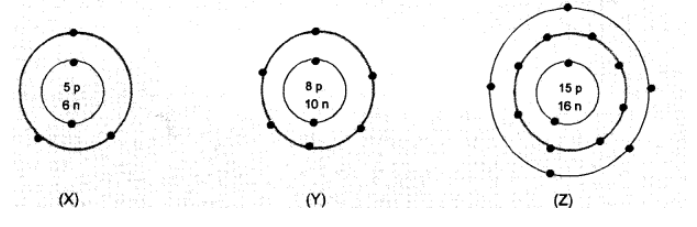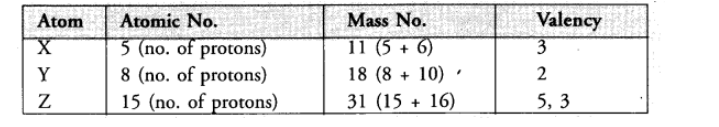# What information do you get from`
Question:

What information do you get from the following electronic distribution about the atomic number, mass number and valency of atoms $X, Y$ and $Z$ ? Give your answer in a tabular form.Solution:- In atom $X$, valency is equal to number of valence electrons $(2,3)=3$

- Atom Y has electronic configuration 2,6 . Valency is eight - No. of valence electrons (8-6)

- In atom Z, valency is equal to number of valence electrons $(2,8,5)$, or eight - no. of valence electrons $(8-5)=3$.